C语言中字符数组作为函数返回值时出现的问题

`````` char *guess_num(int gen_data[], int input_data[], int n)
{
int i = 0, j = 0, k = 0;
static char result[NUM_GUESS];
for(i = 0; i < n; i++)
for(j = 0; j < n; j++)
if(0 == input_data[i]-gen_data[j])
{
if(0 == i-j)
result[k++] = 'A';
else
result[k++] = 'B';
}
if('\0' == result)
for(i = 0; i < n; i++)
result[i] = '0';
return result;
}
``````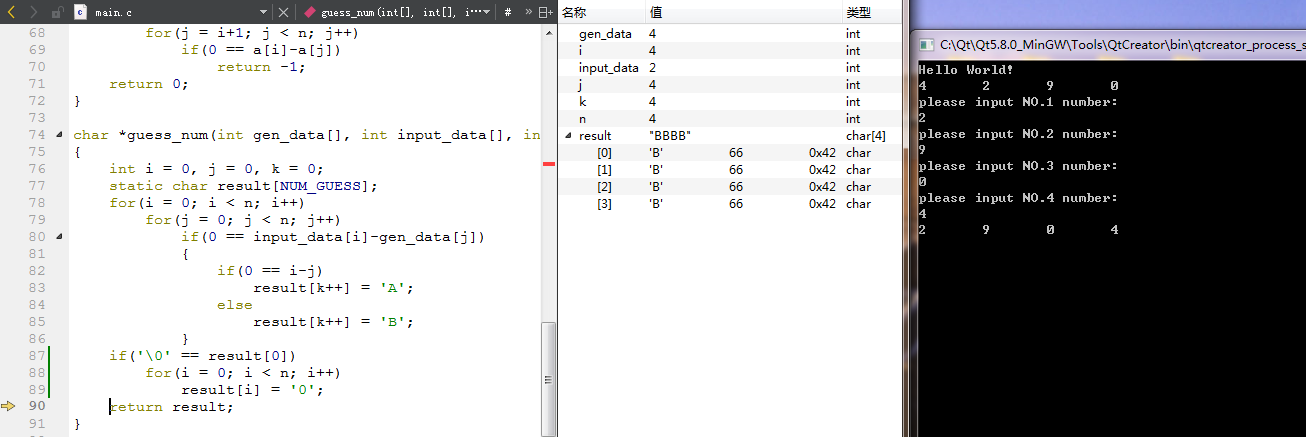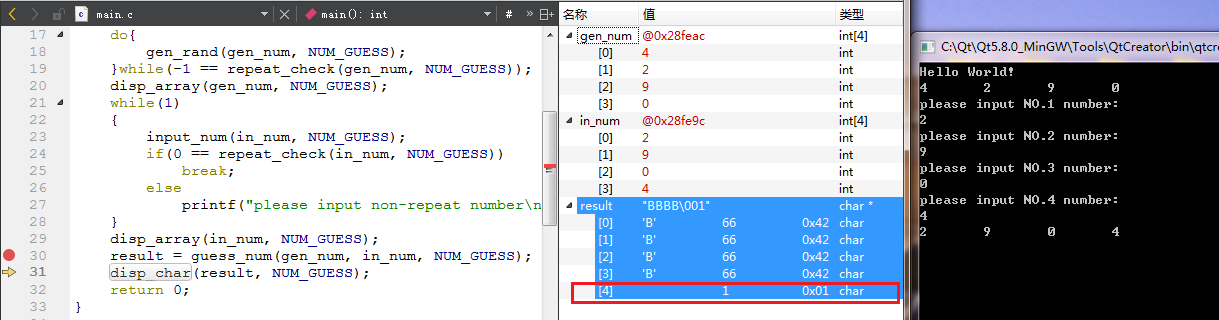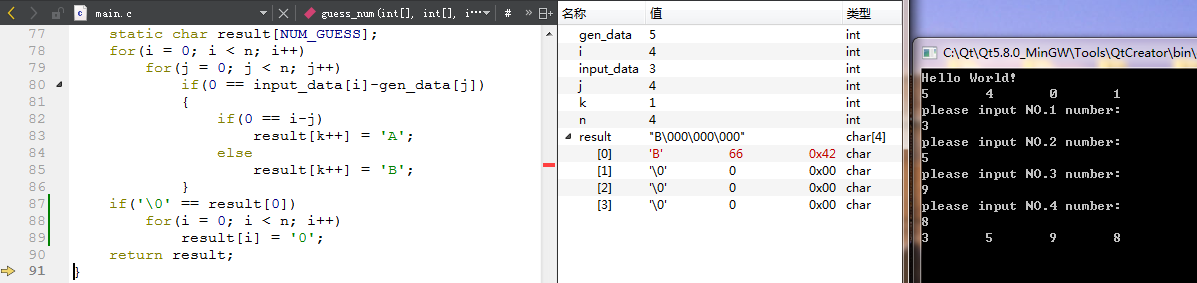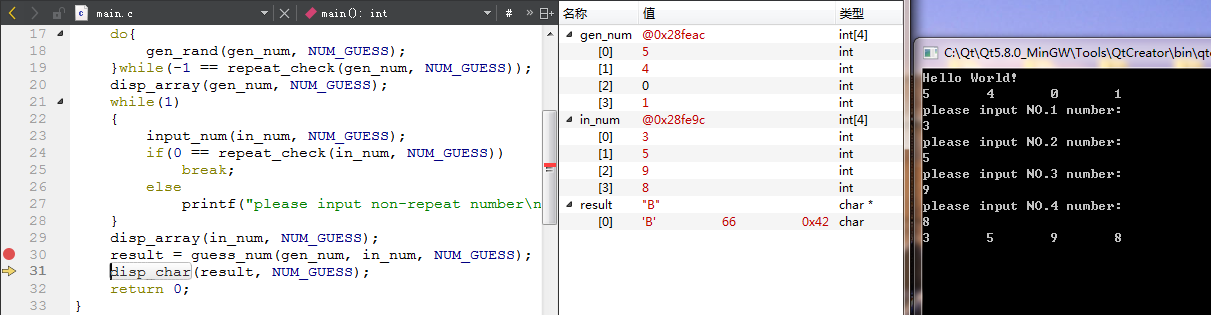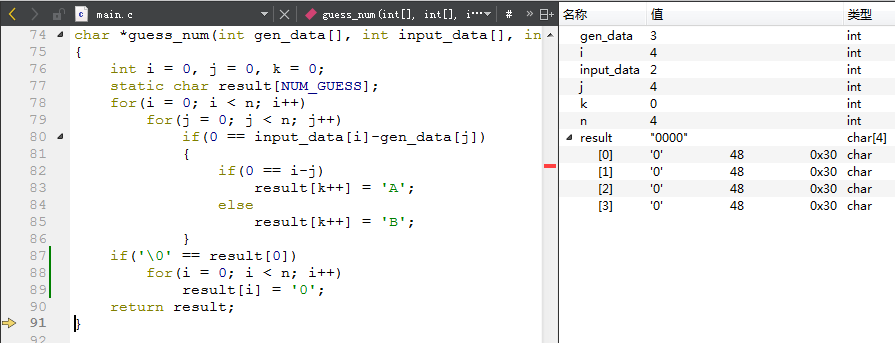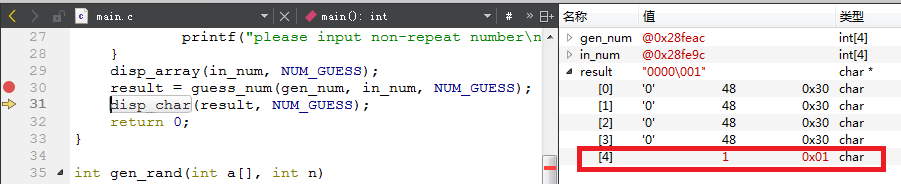5个回答

`````` char *guess_num(int gen_data[], int input_data[], int n)
{
int i = 0, j = 0, k = 0;
static char result = {0};
for(i = 0; i < n; i++)
{
for(j = 0; j < n; j++)
{
if(0 == input_data[i] - gen_data[j])
{
if(0 == i-j)
result[k++] = 'A';
else
result[k++] = 'B';
}
}
if('\0' == result)
{
for(i = 0; i < n; i++)
{
result[i] = '0';
}
}
}
return result;
}
``````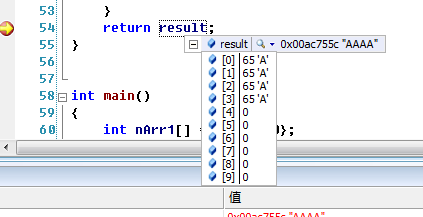WangYun_me 对了，你是申请了10个元素吧？我的数组就4个元素，我的NUM_GUESS是宏定义的，NUM_GUESS=4，所以返回后多出了一个元素，相当于返回后出现了越界，所以我才在这里问这个问题
3 年多之前 回复WangYun_me 你好，你看我的第一张图和第二张图，第一张图和你的一样，在子函数返回的时候，字符数组4个元素都是对的，但是请你看第二张图，当子函数返回后（可以看单步调试的箭头），主函数读取的返回值所指向的地址，数组变成了5个元素，后面多了一个1，图可以放大看，多出的元素我已经标识出来了
3 年多之前 回复WangYun_me 加一个result[k++]那就真的把result这个数组变成5个元素了，我定义的是4个，本来返回后期望得到一个4个元素的数组，但现在情况是返回后得到的数组会多出一个未定义的元素，如果按照你的做法，那就真的变成数组越界了
3 年多之前 回复WangYun_me 不行的，在子函数中定义的数组，并且作为函数返回值，必须使用static将其声明为静态变量，否则子函数返回后，子函数在栈区为变量申请的内存区域会被释放掉，然后主函数还是按照子函数返回的数组首地址取变量，会出现访问越界或者段错误等情况，所以这个static必须要有，不过还是谢谢你
3 年多之前 回复
`````` static char result = {0};  //初始化数据
``````WangYun_me 试过了，和初始化没有关系，数组定义的是4个字符元素，只要是出现4个字符元素全被赋值，返回前都没问题，返回后就会出现在数组后多出一个元素的情况，不过还是谢谢你
3 年多之前 回复